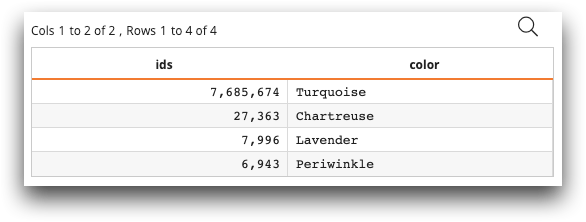# Python in 1010data: Basic syntax

You can use Python to build query operations or ops in the 1010data Macro Language.

The `<code>` tag in Macro Language now supports the attribute `language_="python"`, allowing your analysis to contain Python code. This means that table transformations, query operations, and metadata transformation can now be done with Python. The Python code is run in the accum. See `<code>` in the 1010data Reference Manual for more information about the `<code>` tag.

When you invoke `<code language_="python">`, the platform automatically imports `numpy`, `pandas`, `sklearn`, and `ten`. `numpy` is imported as `np` and `pandas` is imported as `pd`. `ten` is a 1010data-supplied module that is loaded into the Python session. `ten` is used to import data and metadata, get the number of rows, and set to temp tables or worksheets.

On entering the Python session from `<code>`, the session automatically gets the variables `ops` and `table`. `ops` represents the current state of query operations in effect up to the `<code>` tag. `table` is the base table, and will normally be `default.lonely`. On exiting `<code>`, the platform will examine the Python session for a variable named `ops` and extract it. The new `ops` variable will now represent the current state of the query. This allows the user to modify the query by modifying `ops` or replace the query entirely with a completely new set of `ops`.

For example, the end of your Python code can use the `rebase` method in `ten` and then set the value of `ops` as follows:

``ops=ten.rebase(example_data_frame)``

The `DataFrame` `example_data_frame` will then become a worksheet, and on exiting `<code>`, the `DataFrame` will effectively become the new state of the query.

When using the `<code language_="python">` tag, it is good practice to put the Python functions and related operations inside of a `CDATA` tag.

## Syntax

The basic syntax for Python language within 1010data Macro Language is as follows:

``````<code language_="python">
<![CDATA[python code]]>
</code>``````

## Example

The following is a simple example of the Python language within the Macro Language:

``````<base table="default.lonely"/>
<code language_="python">
<![CDATA[
id = np.array([7685674,27363,7996,6943],dtype=np.int32)
col_names = ['Turquoise','Chartreuse','Lavender','Periwinkle']
ops = ten.rebase(pd.DataFrame({'ids':id,'color':col_names}))
]]>
</code>``````

`id` is a NumPy array containing ids. `col_names` is a list containing colors. `ten.rebase` takes `pd.DataFrame` as an argument and returns `ops`. `ops` is a worksheet containing two columns named `ids` and `color`, as shown below:See Macro Language examples for more advanced examples.